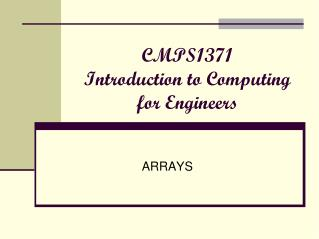Download PresentationCMPS1371 Introduction to Computing for Engineers

# CMPS1371 Introduction to Computing for Engineers - PowerPoint PPT PresentationDownload Presentation## CMPS1371 Introduction to Computing for Engineers

- - - - - - - - - - - - - - - - - - - - - - - - - - - E N D - - - - - - - - - - - - - - - - - - - - - - - - - - -
##### Presentation Transcript

1. ARRAYS CMPS1371Introduction to Computing for Engineers

2. Arrays A “Collection” is a data structure into which you can put items in, and from which you get items out. Arrays are homogeneous collection of things. They are indexable Each element of an array has a value (and a position). A vector is an one dimensional array Big part of Matlabs power is operation on entire Arrays, both on an per element basis and in a “summation” like basis.

3. Matrices: Two Dimensional Arrays “Mat”Lab is all about matrices – originally that's all it was about. A Matrix is a 2-D array.Behave like vectors but two dimensional. “A deck of columns.” As an array, they are indexable and homogeneous

4. Create Array • Remember that we can define a arrays using the following syntax: A= B=[1, 3] or B=[1 3] C=[-1, 0, 0; 1, 1, 0; 0, 0, 2]; With or without commas Semi-colons start a new row

5. Matrix Options • 2-D Matrices can also be entered by listing each row on a separate line C = [-1, 0, 0 1, 1, 0 1, -1, 0 0, 0, 2]

6. These semicolons are optional Matrix Options 2-D matrix

7. You can define a matrix using other matrices as components

8. Array Options • Use an ellipsis to continue a definition onto a new line F = [1, 52, 64, 197, 42, -42, … 55, 82, 22, 109];

9. Index • Indexing a matrix is based on row then column: >> A = [0 3 5 2; 7 6 0 1; 8 2 3 4] >>A(2, 4) ans = 1 2nd row 3rd column

10. Colon Operator • Used to define new matrices • Modify existing matrices • Extract data from existing matrices

11. All the rows in column 1 All the rows in column 4 All the columns in row 1

12. Rows 2 to 3, all the columns You don’t need to extract an entire row or column

13. Rows 2 to 3, in columns 4 to 5

14. A single colon transforms the matrix into a column MATLAB is column dominant

15. Indexing techniques • To identify an element in a 2-D matrix use the row and column number • For example element A(2,3) >> A = [0 3 5 2; 7 6 0 1; 8 2 3 4] >>A(2, 3) ans = 0 2nd row 3rd column

16. Element M(2,3) is in row 2, column 3

17. Or use single value indexing M(8) is the same element as M(2,3) >>M(2, 3) ans = 4 >>M(8) ans = 4 Element #s

18. More Indexing • The word “end” signifies the last element in the row or column Row 1, last element Last row, last element Last element in the single index designation scheme

19. Special Matrices • transpose • The transpose operator changes rows to columns or vice versa. • zeros • Creates a matrix of all zeros • ones • Creates a matrix of all ones • diag • Extracts a diagonal or creates an identity matrix • magic • Creates a “magic” matrix

20. Use the apostrophe to create the transpose The transpose operator makes it easy to create tables

21. With a single input a square matrix is created with the zeros or ones function

22. Two input arguments specify the number of rows and columns

23. The diagonal function When the input argument to the diag function is a square matrix, the diagonal is returned

24. The diagonal function When the input is a vector, it is used as the diagonal of an identity matrix

25. Magic Matrices

26. This woodcut called Melancholia was created by Albrect Durer, in 1514. It contains a magic matrix above the angel’s head

27. Albrect Durer included the date in this magic matrix.